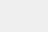# 深度学习框架Keras与Pytorch对比

###### 来源|Towards Data Science#### Keras

Keras本身并不是一个框架，而是一个位于其他深度学习框架之上的高级API。目前它支持TensorFlow、Theano和CNTK。

Keras的优点在于它的易用性。这是迄今为止最容易上手并快速运行的框架。定义神经网络是非常直观的，因为使用API可以将层定义为函数。

#### Pytorch### (1)定义模型的类与函数

[code lang=python]
img_input = layers.Input(shape=input_shape)
x = layers.Conv2D(64, (3, 3), activation='relu')(img_input)
x = layers.Conv2D(64, (3, 3), activation='relu')(x)
x = layers.MaxPooling2D((2, 2), strides=(2, 2))(x)
[/code]

[code lang=python]
class Net(nn.Module):
def __init__(self):
super(Net, self).__init__()
self.conv1 = nn.Conv2d(3, 64, 3)
self.conv2 = nn.Conv2d(64, 64, 3)
self.pool = nn.MaxPool2d(2, 2)
def forward(self, x):
x = F.relu(self.conv1(x))
x = self.pool(F.relu(self.conv2(x)))
return x
model = Net()
[/code]

### (2)张量和计算图模型与标准数组的比较

Keras API向普通程序员隐藏了许多混乱的细节。这使得定义网络层是直观的，并且默认的设置通常足以让你入门。

Pytorch在这些方面更宽容一些。你需要知道每个层的输入和输出大小，但是这是一个比较容易的方面，你可以很快掌握它。你不需要构建一个抽象的计算图，避免了在实际调试时无法看到该抽象的计算图的细节。

Pytorch的另一个优点是平滑性，你可以在Torch张量和Numpy数组之间来回切换。如果你需要实现一些自定义的东西，那么在TF张量和Numpy数组之间来回切换可能会很麻烦，这要求开发人员对TensorFlow会话有一个较好的理解。

Pytorch的互操作实际上要简单得多。你只需要知道两种操作:一种是将Torch张量(一个可变对象)转换为Numpy，另一种是反向操作。

### (3)训练模型[code lang=python]
history = model.fit_generator(
generator=train_generator,
epochs=10,
validation_data=validation_generator)
[/code]

1. 在每批训练开始时初始化梯度
2. 前向传播
3. 反向传播
4. 计算损失并更新权重

[code lang=python]
# 在数据集上循环多次
for epoch in range(2):
for i, data in enumerate(trainloader, 0):
# 获取输入; data是列表[inputs, labels]
inputs, labels = data
# (1) 初始化梯度

# (2) 前向传播
outputs = net(inputs)
loss = criterion(outputs, labels)

# (3) 反向传播
loss.backward()
# (4) 计算损失并更新权重
optimizer.step()
[/code]

###　(4)控制CPU与GPU模式的比较[code lang=python]
with tf.device('/cpu:0'):
y = apply_non_max_suppression(x)
[/code]

[code lang=python]
#获取GPU设备
device = torch.device("cuda:0" if torch.cuda.is_available() else "cpu")

#传送网络到GPU
net.to(device)

# 传送输入和标签到GPU
inputs, labels = data.to(device), data.to(device)
[/code]

Keras在这方面的优势在于它的简单性和良好的默认设置

### 选择框架的一般建议

Keras绝对是最容易使用、理解和快速上手并运行的框架。你不需要担心GPU设置，处理抽象代码，或者做任何复杂的事情。你甚至可以在不接触TensorFlow的任何一行的情况下实现定制层和损失函数。

Keras 教程：http://keraschina.com
PyTorch 教程：http://pytorch123.com